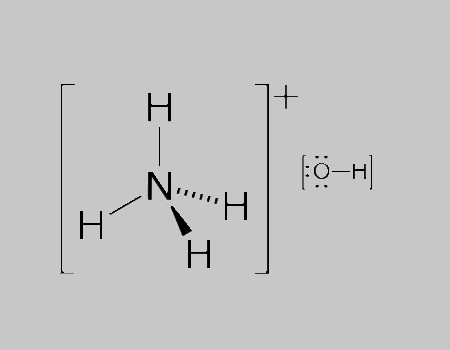# Ammonium Hydroxide Formula

Ammonium Hydroxide formula is a topic of constant debate among many. It is because Ammonium hydroxide is basically a solution of ammonia in water. Even though the name suggests an alkali with the composition of NH4+ and OH− ions, in practicality it is impossible to isolate samples of ammonium hydroxide. Furthermore, the ions also do not account for a significant fraction of the total amount of ammonia.

## Ammonium Hydroxide Chemical Formula

The accepted chemical formula of ammonium hydroxide is NH4OH where ammonium has a charge of +1 and hydroxide has a charge of -1. When they react they both cancel each other out and balance automatically in the end.

However, the water solution of ammonia is represented by the formula even though no significant amount of the molecular species NH4OH is found. Instead, the solution contains a mixture of water (H2O) and ammonia (NH3) in large quantities while ammonium and hydroxide ions are found in smaller quantities. Ammonia also deprotonates some of the water.

 Formula NH4OH or NH5O Molar Mass 35.05 g/mo Density 0.91 g/cm3 Melting Point −57.5°C Boiling Point 37.7 °C

### Ammonium Hydroxide Structural Formula

Ammonium hydroxide, also known as ammonia water, ammonia solution, ammoniacal liquor, ammonia liquor, aqua ammonia, aqueous ammonia. A representation of oxygen structural formula is given below.Do stay tuned to BYJU’S to access pages of different formulas of important chemical compounds.